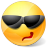# Math Is Fun Forum

Discussion about math, puzzles, games and fun.   Useful symbols: ÷ × ½ √ ∞ ≠ ≤ ≥ ≈ ⇒ ± ∈ Δ θ ∴ ∑ ∫  π  -¹ ² ³ °

You are not logged in.

## #1 2009-01-22 07:57:54

careless25
Real MemberRegistered: 2008-07-24
Posts: 560

### Asymptotes and Slants

I just need a simple explanation for how horizontal asymptotes work?
and what makes slants?

Vertical asymptotes are just denominator equal to 0. to solve for x.

f(x)= (x+3)/(x-1)
V.A. x = 1
H.A. y = ?
No slant cause you cannot factor?

Offline

## #2 2009-01-22 08:08:33

mathsmypassion
Member
Registered: 2008-12-01
Posts: 33

### Re: Asymptotes and Slants

To find horizontal asymptotes you have to calculate the limits to +  ∞ and - ∞. If any of them is a finite number, that's a HA. In your case, both limits are 1 so y = 1 is HA (to + ∞ and - ∞).

For slants: if a function does not have HA, it may have slants asymptotes.
For example: f(x) = (x^2 + 1)/(x + 1)
In order to find the SA follow the steps:
1. calculate the limit to +∞ for f(x)/x. If this limit if finite (a real number ≠ 0), let's call it m, than m would represent the slope of the SA. Go to step 2. If the limit is not finite , the function does not have SA to +∞. Go to step 4.
2. Find now the limit to +∞ for f(x) - mx and call this real number n.
3. The SA is y = mx + n
4. Do the same thing for  -∞ . You might get a different asymptote.

Last edited by mathsmypassion (2009-01-22 08:51:16)

Offline